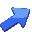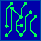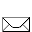# Linear Programming - Simplex Applet

By Pedro Miguel Silva and Tiago Castro Guise
Version 1.0 - Lisbon, July 1998, updated on October 1999

You are visitor numberto this page since last reboot.

### Simplex Applet:

The available LP algorithms are: Simplex Method, Revised Method, Primal Dual and Simplex Dual.

###Enter Your Linear Program:

`... Applet Controls here, if you had java in your browser. `

### How the Applet Works:

Buttons:
• Solve - Solve your linear program.
• Abort - Abort the execution of the algorithm.
• Clear - Allows you to clear fields.
• First Choice Menu - With this options you can chose clear the field results or clear the field linear program. It is also, available the options of  no clear fields and clear all fields.
• Second Choice Menu - Chose the algorithm you want Simplex, Revised Simplex, Primal Dual or Simplex Dual. .
• Third Choice Menu - Chose output options.

### Linear Programming:

A linear program is a problem a problem that can be expressed as follows:

min          cx          (Standard Form)
subject to Ax = b
x >= 0

Where "x" is the vector of variables to be solved, "A" is the matrix of known coefficients and "c" and "b" are vectors of known coefficients. The Expression "cx" is called the objective function and  the equations "Ax = b" are called the constraints.

### Syntax of Linear Program:

[max:|min:]   c1 x1   + ... + cN xN;   (Objective function)
[c1:]   a11x+ ... + a1NxN    "= | > | < | => | <=" b1;   (Constraints)
[cM:]  aM1x1 + ... + aMNx"= | > | < | => | <=" bM;

Where "x" is the vector of variables to be solved for, "A" is a matrix of known coefficients, and "c" and "b" are vectors of known coefficients.  M is the number of constraints and N is the number of variables.

### A Small Example:

The following example demonstrates the applet. This problem requires the two phases method.

2 constraints and 2 variables

min: x1 + 1.5 x2;
c1: 0.5 x1 + x2 >= 7.5;
2 x1 + x2 >= 15;
x1 >= 0;
x2 >= 0;INESC
Instituto de Engenharia de Sistemas e Computadores
Lisbon, Portugal - Europe
ALGOS Group ALGorithms for Optimization and Simulation
Created by: Pedro Miguel Silva and Tiago Castro GuiseGive us your  Comment .